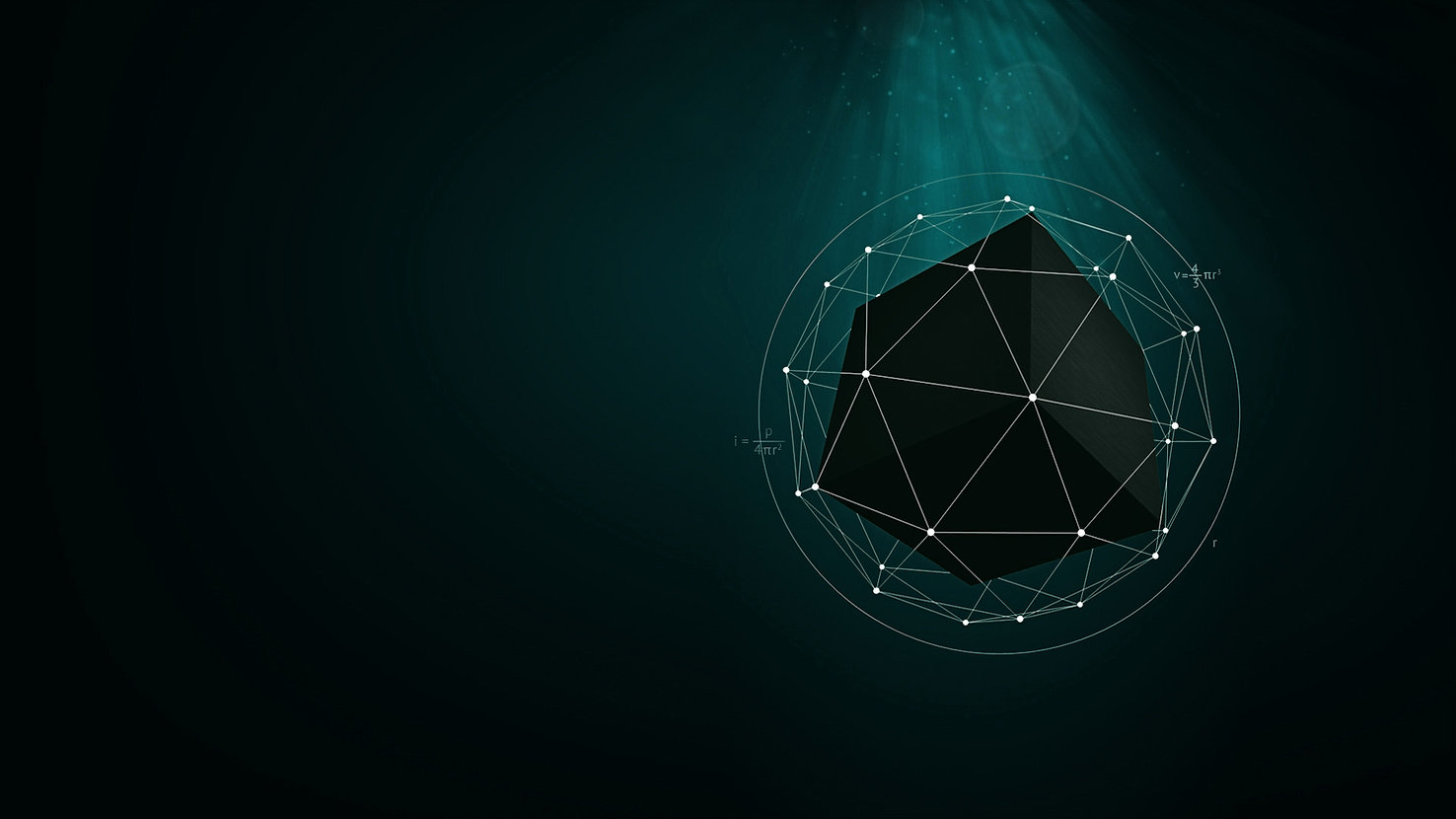G-C8J3SKTJETMath, short for Mathematics is the comprehensive science of numbers and their operations, interrelations, combinations, generalizations, abstractions,  space configurations and their structure, measurement, transformations, and generalizations.

It is the science of structure, order, and relation that has evolved from counting, measuring, and describing the shapes of objects. It deals with logical reasoning and quantitative calculation. It has long been an indispensable adjunct to the physical sciences and technology, to the extent that it is considered the underlying language of science. Among the principal branches of mathematics are algebra, analysis, arithmetic, combinatorics, Euclidean and non-Euclidean geometries, game theory, number theory, numerical analysis, optimization, probability, set theory, statistics, topology, and trigonometry.

## The Hall of the Studies of the Nature of Mathematics

Ah mathematics, in this great hall you will learn how the ancients invented & integrated mathematics into their daily lives. You will learn from the foundation about mathematics and how to become an expert in math.